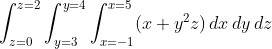## Triple Integrals

Evaluate the triple integral:Hint
Since the order of integration is specified in the problem, integrate with respect to $$x$$ first, then $$y$$ , and then finally $$z$$ .
Hint 2
$$\int x^n \:dx=\frac{x^{n+1}}{n+1}$$$Since the order of integration is specified in the problem, integrate with respect to $$x$$ first, then $$y$$ , and then finally $$z$$ . $$\int_{z=0}^{z=2}\int_{y=3}^{y=4}\int_{x=-1}^{x=5}(x+y^2z)\, dx\, dy\, dz$$$
Recall the power rule:
$$\int x^n \:dx=\frac{x^{n+1}}{n+1}$$$Integrate with respect to $$x$$ : $$=\int_{z=0}^{z=2}\int_{y=3}^{y=4}\frac{x^2}{2}+xy^2z \bigg\rvert_{x=-1}^{x=5}\, dy\, dz$$$
$$=\int_{z=0}^{z=2}\int_{y=3}^{y=4}\frac{5^2}{2}+5y^2z-\frac{(-1)^2}{2}-(-1)y^2z \: dy\, dz$$$$$=\int_{z=0}^{z=2}\int_{y=3}^{y=4}12.5+5y^2z-0.5+1y^2z \: dy\, dz$$$
$$=\int_{z=0}^{z=2}\int_{y=3}^{y=4}12+6y^2z \: dy\, dz$$$Integrate with respect to $$y$$ : $$=\int_{z=0}^{z=2}12y+\frac{6y^3z}{3} \bigg\rvert_{y=3}^{y=4} \: dz$$$
$$=\int_{z=0}^{z=2}12(4)+2(4)^3z-12(3)-2(3)^3z \: dz$$$$$=\int_{z=0}^{z=2}48+128z-36-54z \: dz=\int_{z=0}^{z=2}12+74z \: dz$$$
Integrate with respect to $$z$$ :
$$=12z+\frac{74z^2}{2} \bigg\rvert_{z=0}^{z=2}=12(2)+37(2)^2-12(0)-37(0)^2$$$$$=24+148-0-0=172$$$
172